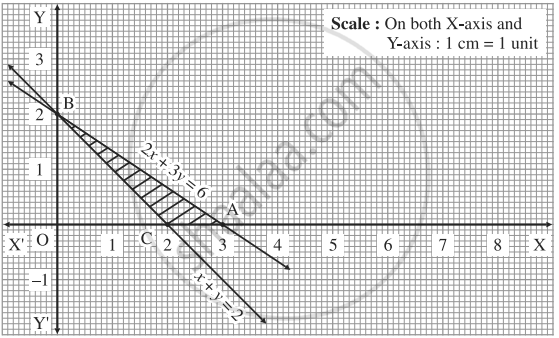Advertisement Remove all ads

# Find the feasible solution of the following inequation: 2x + 3y ≤ 6, x + y ≥ 2, x ≥ 0, y ≥ 0 - Mathematics and Statistics

Sum

Find the feasible solution of the following inequation:

2x + 3y ≤ 6, x + y ≥ 2, x ≥ 0, y ≥ 0

Advertisement Remove all ads

#### Solution

First we draw the lines AB and CB whose equations are 2x + 3y = 6 and  x + y = 2 respectively.

 Line Equation Points on the X-axis Points on the Y-axis Sign Region AB 2x + 3y = 6 A (3,0) B (0,2) ≤ origin side of line AB CB x + y = 2 C (2,0) D(0,2) ≥ non -origin side of line CBThe feasible solution is Δ ABC which is shaded in the graph.

Concept: Linear Programming Problem (L.P.P.)
Is there an error in this question or solution?
Advertisement Remove all ads

#### APPEARS IN

Advertisement Remove all ads
Advertisement Remove all ads
Share
Notifications

View all notifications

Forgot password?